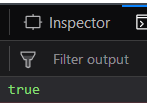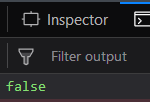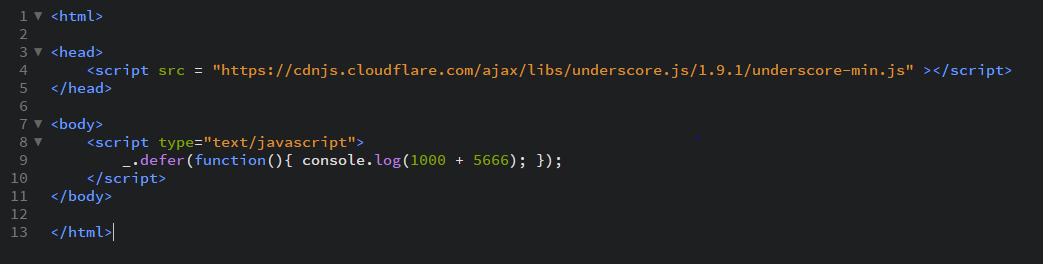# Underscore.js _.isElement() Function

• Last Updated : 25 Nov, 2021

The _.isElement() function: is used to check whether the element is a document object model or not. A document object model is the way javascript sees the data of the containing pages. The Cascading style sheet (CSS) and javascript (JS) interact with Document object model (DOM).

Syntax:

`_.isElement(object)`

Parameters:
It takes only one argument which is the object element that needs to be checked.

Return value:
It returns the true if it is a DOM element otherwise returns false.

Examples:

1. Passing html tag to the _.isElement() function:
The _.isElement() function takes the element and perform the checking function. It checks whether it is a DOM element or not. Like here, the argument passed to the _.isElement() function is ‘html’ and since we know it is a DOM element so, true is returned in the output.
 ```<``html``>``  ` `<``head``>``    ``<``script` `src` `= ``    ``"https://cdnjs.cloudflare.com/ajax/libs/underscore.js/1.9.1/underscore-min.js"``>``     ```` ` `    ``<``script` `src``=``    ``"https://ajax.aspnetcdn.com/ajax/jQuery/jquery-3.3.1.min.js"``>``    ``````  ` `<``body``>``    ``<``script` `type``=``"text/javascript"``>``        ``console.log(_.isElement(jQuery('html')));``    ``````  ` ``

Output:2. Passing body tag to the _.isElement() function:
In this case, we pass ‘body’ tag as an argument to the _.isElement() function. Since, we know that ‘body’ tag is a DOM element therefore the output will be true.
 ```<``html``>``  ` `<``head``>``    ``<``script` `src` `= ``    ``"https://cdnjs.cloudflare.com/ajax/libs/underscore.js/1.9.1/underscore-min.js"``>``    ```` ` `    ``<``script` `src``=``    ``"https://ajax.aspnetcdn.com/ajax/jQuery/jquery-3.3.1.min.js"``>``    ``````  ` `<``body``>``    ``<``script` `type``=``"text/javascript"``>``        ``console.log(_.isElement(jQuery('body')));``    ``````  ` ``

Output:3. Passing div tag to the _.isElement() function:
In this case, we pass ‘div’ tag as an argument to the _.isElement() function. Since, we know that ‘div’ tag is a DOM element therefore the output will be true.
 `<``html``>``  ` `<``head``>``    ``<``script` `src` `= ``    ``"https://cdnjs.cloudflare.com/ajax/libs/underscore.js/1.9.1/underscore-min.js"``>``    ````    ``<``script` `src``=``    ``"https://ajax.aspnetcdn.com/ajax/jQuery/jquery-3.3.1.min.js"``>``    ``````  ` `<``body``>``    ``<``script` `type``=``"text/javascript"``>``        ``console.log(_.isElement(_.isElement(jQuery('div'))));``    ``````  ` ``

Output:4. Using and (&&) operation in the _.isElement() function:
We can even use 2 _.isElement() functions to obtain the output like in the below example. First, both of their answers will be calculated and then an ‘and’ operation will be performed. AND operation gives true only if both the answers are true, otherwise it will give false as answer.
 ``` ` `<``html``>``  ` `<``head``>``    ``<``script` `src` `= ``    ``"https://cdnjs.cloudflare.com/ajax/libs/underscore.js/1.9.1/underscore-min.js"``>``     ```` ` `    ``<``script` `src``=``    ``"https://ajax.aspnetcdn.com/ajax/jQuery/jquery-3.3.1.min.js"``>``    ``````  ` `<``body``>``    ``<``script` `type``=``"text/javascript"``>``     ``console.log(_.isElement(jQuery('html')) && _.isElement(jQuery('div')));``     ``````  ` ``

Output:`

NOTE:
These commands will not work in Google console or in firefox as for these additional files need to be added which they didn’t have added.
 ```<``script` `type``=``"text/javascript"` `src` `=``"https://cdnjs.cloudflare.com/ajax/libs/underscore.js/1.9.1/underscore-min.js"``>```` ` ```<``script` `src``=``"https://ajax.aspnetcdn.com/ajax/jQuery/jquery-3.3.1.min.js"``>```